# Gradients of Real Life Graphs

GCSEKS3Level 4-5Level 6-7AQACambridge iGCSEEdexcelEdexcel iGCSEOCRWJEC

## Real Life Graphs

Real life graphs are graphs representing real things, these can be straight line graphs and curved graphs.

These graphs can represent anything so getting the basics is important.

Make sure you are happy with the following topics before continuing:

Level 4-5Level 6-7GCSEAQAEdexcelOCRWJECCambridge iGCSEEdexcel iGCSE

## Gradient Represents a Rate of Change

For many real life graphs the gradient will represent the rate.
Take a look at the examples shown.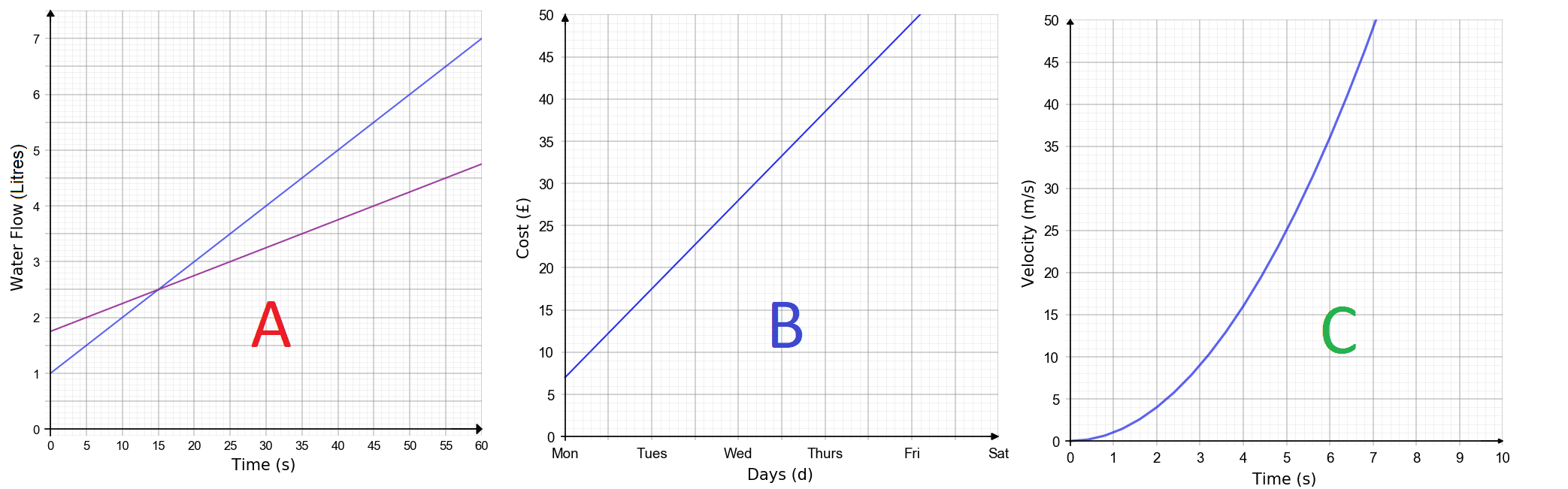What rate does each graph represent?

A: Gradient = Litres PER Second (Rate of flow)

B: Gradient = Cost PER Day (Price per day)

C: Gradient = Metres per second PER second (Acceleration)

Note: C is a curved graph so the gradient changes over time, this means we have to use some other techniques for finding the gradient.

Level 4-5GCSEKS3AQAEdexcelOCRWJECCambridge iGCSEEdexcel iGCSE
Level 6-7GCSEAQAEdexcelOCRWJECCambridge iGCSEEdexcel iGCSE

You may be asked to find the average gradient between two points.

Example: Sammy is running a $100$ m race. His distance from the finish line is plotted on the distance time graph shown.

Calculate his average speed between $4$ seconds and $12$ seconds.

Step 1:  Draw a line between the two points on the graph at $4$ seconds and $12$ seconds, shown on the graph below.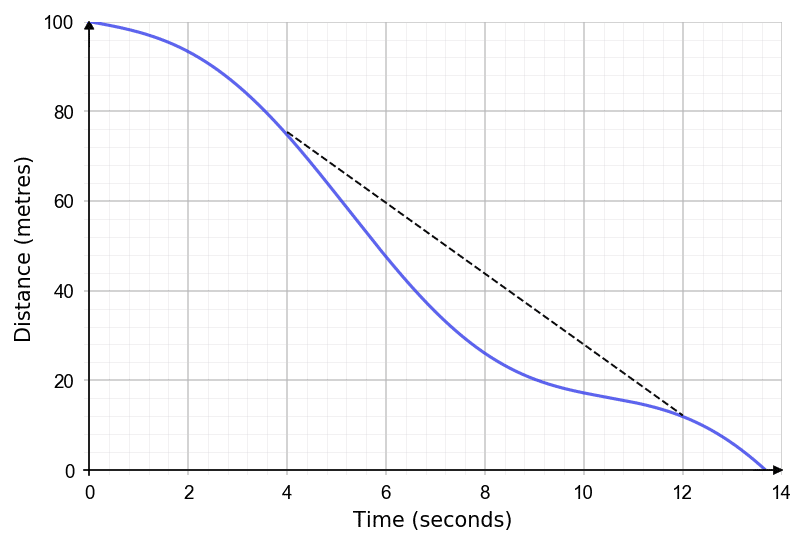Step 2: Find the gradient of the straight line.

$\text{Gradient} = \dfrac{\text{Change in }y}{\text{Change in }x} = \dfrac{76-12}{12-4} = \dfrac{64}{8} = 8$ m/s

Level 6-7GCSEAQAEdexcelOCRWJECCambridge iGCSEEdexcel iGCSE

## Estimating Rate at a Given Point

We calculate the instantaneous rate of change by drawing a tangent to the curve (a straight line just touching the curve) at the desired point, and then calculating the gradient of this tangent (which can be worked out using standard straight line methods).

This will correspond to the gradient of the curve at that individual point.

Example: The descent of a high altitude weather balloon from $40$ km above sea level is shown on the graph shown.

Work out the rate of descent at after $5$ minutes.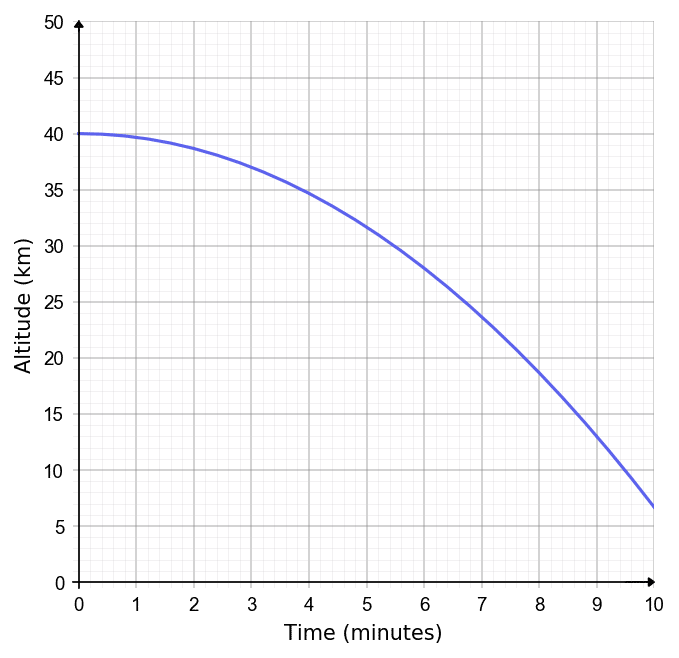To find the descent rate, we can draw a tangent line to the graph at that point and calculate its gradient. We can form a triangle with the hypotenuse being the tangent to the line at $x=5$ ($5$ minutes in). This can be seen below.

Hence,

$\dfrac{\text{Change in } y}{\text{Change in } x}= \dfrac{45-18.5}{9-1} =3.31$ km/min ($2$ dp).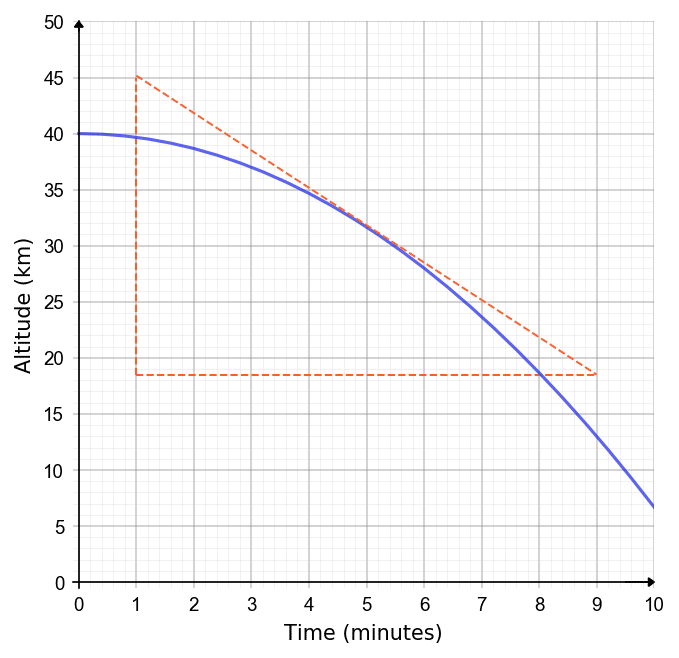Level 6-7GCSEAQAEdexcelOCRWJECCambridge iGCSEEdexcel iGCSE

## Example 1: Gradient of a Real-Life Graph

Richmondshire Cars is a taxi company that charge a base rate per journey then a certain amount per mile. Use the graph below to calculate:

i) The base rate charged per journey.

ii) The amount charged per mile.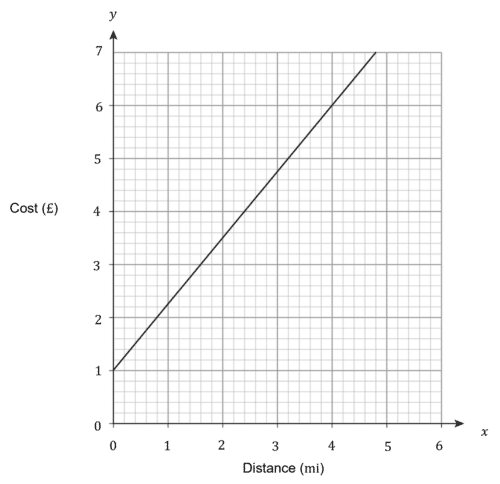[3 marks]

i) At $0$ miles, the cost is $£1$, so this must be the base rate per journey.

ii) The cost per mile is the gradient.

$\text{Cost per mile}=\dfrac{7-1}{4.8-0}=\dfrac{6}{4.8}=£1.25$

Level 4-5GCSEAQAEdexcelOCRWJECCambridge iGCSEEdexcel iGCSE

## Example 2: Instantaneous Rate of Change

Jamie is making popcorn. He records the pops he hears from the pan over $70$ seconds.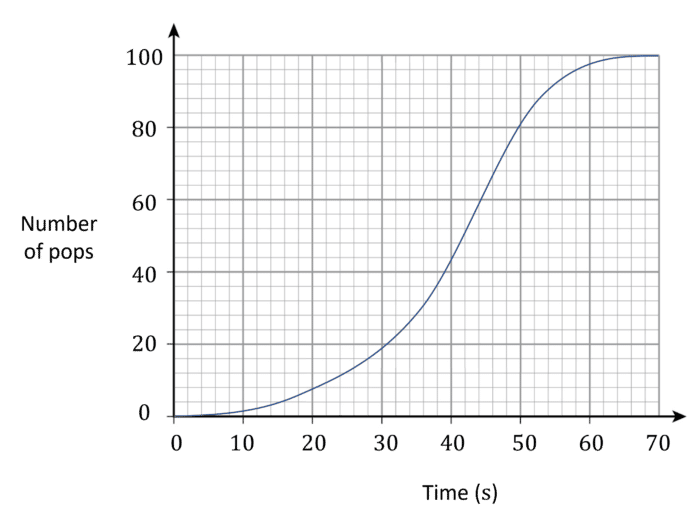i) Find the average rate of pops at $40\text{ s}$.

ii) By drawing a tangent, or otherwise, find the instantaneous rate of pops at $40\text{ s}$.

[3 marks]

i) At $40\text{ s}$ there have been $44$ pops, for an average of $\dfrac{44}{40}=1.1$ pops per second.

ii) Tangent passes through $(40,44)$ and $(50,80)$.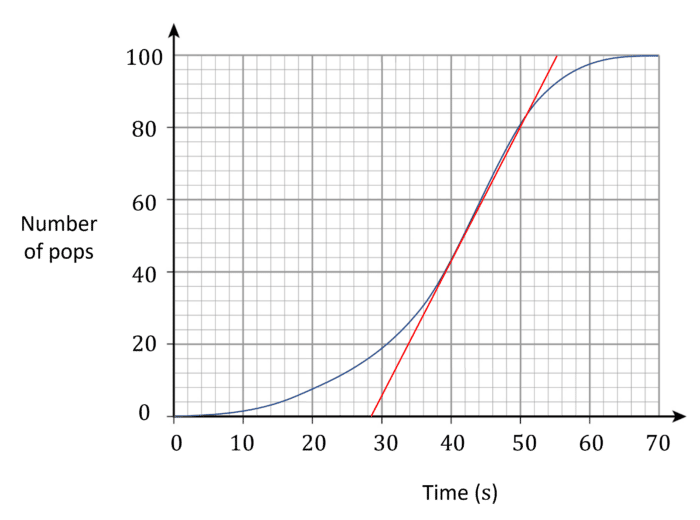Gradient $=\dfrac{80-44}{50-40}=\dfrac{36}{10}=3.6$ pops per second.

Level 6-7GCSEAQAEdexcelOCRWJECCambridge iGCSEEdexcel iGCSE

## Gradients of Real Life Graphs Example Questions

To find each cost corresponding to $40$ litres, first locate $40$ litres on the $x$-axis and draw a straight line up to both lines. Next draw a line straight across to the $y$-axis.

$\text{Diesel cost} \approx £46.00$

$\text{Petrol cost} \approx £40.50$

Hence the difference in price for a full $40$ litre tank of fuel is,

$£46.00 - £40.50 = £5.50$Gold Standard Education

To find the cost of a single hire, locate Thursday on the $x$-axis and draw a straight line upwards to meet the line. Tracing across to the $y$-axis we find,

$\text{Cost per bike} \approx £38.00$

As the group is looking to hire $4$ bikes, multiple the cost by four,

$\text{Total cost } = £38.00 \times 4 = £152$Gold Standard Education

i) The cost at $0$ hours is the cost of the materials only. This is $£2600$

ii) Find gradient by choosing two points e.g. (0,2600) and (60,5000).

$\text{Gradient}=\dfrac{5000-2600}{60-0}=\dfrac{2400}{60}=£40$

Hence, Emma charges $£40$ per hour.Gold Standard Education

To find the acceleration at $5$ seconds after launch we need to find the gradient of the line at that point. To find the gradient we can draw a large triangle with the hypotenuse being the tangent to the line at $x=5$. This can be seen below.

Hence,

$\dfrac{\text{Change in } y}{\text{Change in } x}= \dfrac{45-5}{7-3} =10$ m/s$^2$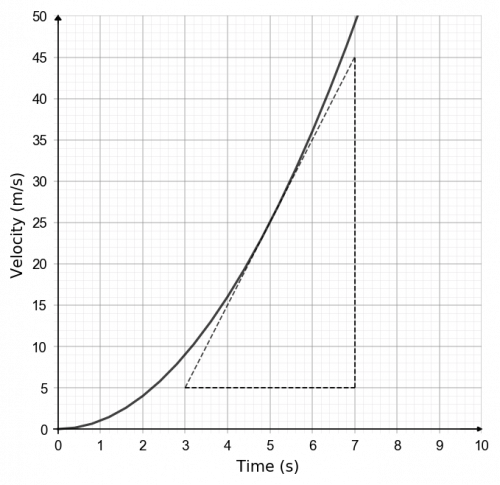Gold Standard Education

## Gradients of Real Life Graphs Worksheet and Example Questions

### (NEW) Real Life Graphs Exam Style Questions

Level 4-5Level 6-7GCSENewOfficial MMEGold Standard Education

£19.99 /month. Cancel anytime

Level 4-5GCSE